U.S. Department of Transportation
1200 New Jersey Avenue, SE
Washington, DC 20590
202-366-4000

Federal Highway Administration Research and Technology
Coordinating, Developing, and Delivering Highway Transportation InnovationsThis report is an archived publication and may contain dated technical, contact, and link information
 Federal Highway Administration > Publications > Research Publications > 04097 > Measured Variability Of Southern Yellow Pine - Manual for LS-DYNA Wood Material Model 143
Publication Number: FHWA-HRT-04-097
Date: August 2007

# Measured Variability Of Southern Yellow Pine - Manual for LS-DYNA Wood Material Model 143

PDF files can be viewed with the Acrobat® Reader®

### APPENDIX E. DERIVATION OF CONSISTENCY PARAMETER FOR PLASTICITY ALGORITHM

Plasticity is modeled by enforcing separate consistency conditions for the parallel and perpendicular modes. The goal of each consistency condition is to partition the total strain increments into elastic and plastic components:

The superscripts e and p indicate the elastic and plastic components, respectively. The total strain increments (Deij) are calculated by the finite element code from the dynamic equations of motion and the time step. Once this partition is known, then the stress increments are updated from the elastic strain increments:

Here, n denotes the nth time step in the finite element analysis.

### E.1 CHECK FOR YIELDING

The partition into elastic and plastic components requires two steps. The first step is to check for yielding. This is done by temporarily updating the stress components from the incremental strains by assuming that the entire strain increment is elastic:

These updated stresses are called the trial elastic stresses (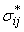). The trial elastic stress invariants (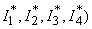are updated from the trial elastic stresses. The value of the yield function is evaluated from the trial elastic invariants and is denoted as f *. The new stress state is elastic if * ≤ 0 and plastic if * > 0.

### E.2 CALCULATE CONSISTENCY PARAMETER

The second step is to enforce the consistency condition if *> 0. Enforcement of the consistency condition requires an assumption about the direction of plastic flow. It is assumed that the plastic strain increments are normal to the yield surface:

where Dl is a proportionality constant known as the consistency parameter.

This assumption is known as an associated flow rule, or normality condition. Use of a potential function other than the yield function in equation 180 results in a nonassociated flow rule. Recent studies reported by Pucik(35) suggest that rate-independent models with nonassociated flow lead to spurious (non-unique) dynamic solutions, so only associated flow is proposed for the present model.

The plasticity algorithm calculates Dl by enforcing the plastic consistency condition. This condition is expressed as:

where f is the yield surface function at time increments n to n + 1. The stress state at the beginning of the time step lies on the yield surface, thus, f n = 0. The stress state at the end of the time step is returned to the yield surface by the plasticity algorithm, thus, f n+1 = 0. Therefore, Df = 0.

The solution of the consistency condition in equation 181 determines Dl, which, in turn, determines the partitioning of the total strain rate into elastic and plastic components. The stresses are updated from the elastic strain components. Separate Dl solutions are proposed for the parallel and perpendicular modes.

Parallel Modes

The consistency condition is derived in terms of the invariants rather than the stresses, because the parallel failure criterion in equation 13 is formulated in terms of two transversely isotropic stress invariants. For the purposes of this derivation, the parallel failure criterion from equation 13 is defined as f||(I1,I4) ≥ 0, with:

A first-order Taylor series expansion of the consistency condition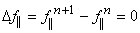from time increment n to n + 1 gives:

Expansion of the stress invariant increments gives:

where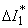and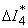are the trial elastic increments calculated with Dl|| = 0. Substitution of the updates from equations 184 and 185 into the consistency condition in equation 183 results in the following expression for Dl||:

The expression in the numerator is recognized as the first-order Taylor series expansion of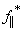, where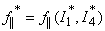is the value of the failure criterion calculated from the trial elastic invariants. Therefore, the expression for Dl|| reduces to:

Perpendicular Modes

The perpendicular failure criterion in equation 14 is derived in terms of the invariants rather than the stresses. The perpendicular failure criterion is defined as f^(I2,I3) = 0, with:

Expansion of the consistency condition Df^ = 0 gives: